## Lesson 12. Rheological properties measurement and equipment’s

A viscometer (also called viscometer) is an instrument used to measure the viscosity of a fluid. For liquids with viscosities which vary with flow conditions, an instrument called a rheometer is used. Viscometers only measure under one flow condition

In general, either the fluid remains stationary and an object moves through it, or the object is stationary and the fluid moves past it. The drag caused by relative motion of the fluid and a surface is a measure of the viscosity. The flow conditions must have a sufficiently small value of Reynolds number for there to be laminar flow.

Methods for measuring viscosity:

1. Capillary type:

The device is also known as U-tube viscometer or Ostwald viscometers, named after Wilhelm Ostwald. The time for a standard volume of fluid to pass through a length of capillary tubing is measured. This type of flow is described by Poiseuille equation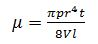µ= fluid viscosity p= driving pressure r= radius of capillary t= time of flow V= volume of flow l= length of capillary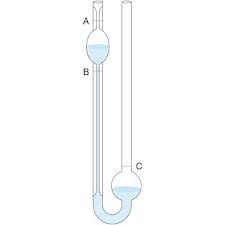Another version is the Ubbelohde viscometer, which consists of a U-shaped glass tube held vertically in a controlled temperature bath. In one arm of the U is a vertical section of precise narrow bore (the capillary). Above this is a bulb, with it is another bulb lower down on the other arm. In use, liquid is drawn into the upper bulb by suction, then allowed to flow down through the capillary into the lower bulb. Two marks (one above and one below the upper bulb) indicate a known volume. The time taken for the level of the liquid to pass between these marks is proportional to the kinematic viscosity. Most commercial units are provided with a conversion factor, or can be calibrated by a fluid of known properties. The time required for the test liquid to flow through a capillary of a known diameter of a certain factor between two marked points is measured. By multiplying the time taken by the factor of the viscometer, the kinematic viscosity is obtained.

2. Orifice type:

This can be considered as a very short capillary type viscometer. The time for a standard volume of fluid to flow through an orifice is measured. This is a simple, inexpensive rapid method that is widely used in quality control of Newtonian and non-Newtonian fluids where extreme accuracy is not needed.

This consists of a stainless –steel 44 ml capacity cup attached to a handle with a calibrated circular hole in the bottom. In operation the cup is filled by dipping into the fluid and withdrawing it. A stopwatch is started as soon as it is withdrawn and stopped when the first break occurs in the issuing stream; the elapsed time gives an empirical value of viscosity.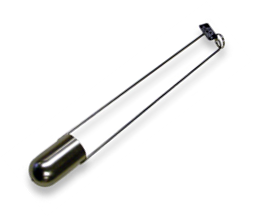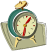3. Coaxial rotational viscometer:

These are also known as concentric cylinder viscometer. A spindle having circular cross section is placed inside a cup containing the test liquid. Either the cup or the spindle is rotated and the drag of the fluid on the spindle is measured by means of  torque sensor. It can be used for both Newtonian and non-Newtonian fluids and this type is widely used in food industry Eg. Haake rotoviscometer, rheometers,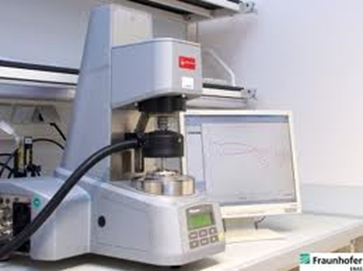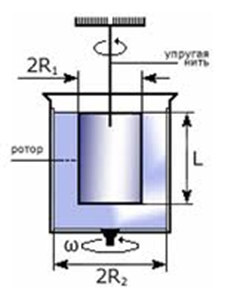4.  Cone and plate viscometer:

The fluid is held by its own surface tension between a cone of small angle that just touches a flat surface. The torque is caused by the drag of the fluid on the cone is measured as one of the members is rotated while the other member remains stationary.

For a Newtonian fluid following equation applies: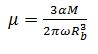Where, µ= fluid viscosity a= angle of cone, 2° M= torque w=angular velocity Rb = radius of cone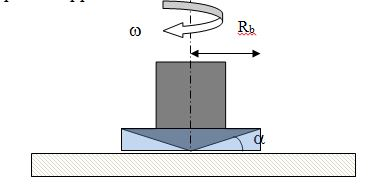5.  Falling ball viscometers

Stokes' law is the basis of the falling sphere viscometer, in which the fluid is stationary in a vertical glass tube. A sphere of known size and density is allowed to descend through the liquid. If correctly selected, it reaches terminal velocity, which can be measured by the time it takes to pass two marks on the tube. Knowing the terminal velocity, the size and density of the sphere, and the density of the liquid, Stokes' law can be used to calculate the viscosity of the fluid.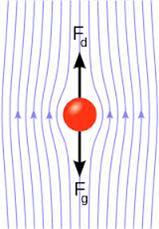Fig.  Falling sphere

In 1851, George Gabriel Stokes derived an expression for the frictional force (also called drag force) exerted on spherical objects with very small Reynolds numbers (e.g., very small particles NRe<1) in a continuous viscous fluid by changing the small fluid-mass limit of the generally unsolvable Navier-Stokes equations:

where:

FD= frictional force,

r= radius of the spherical object,

m= fluid viscosity, and

v =particle's velocity.

If the particles are falling in the viscous fluid by their own weight, then a terminal velocity, also known as the settling velocity, is reached when this frictional force combined with the buoyant force exactly balance the gravitational force. The resulting settling velocity (or terminal velocity) is given by: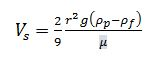where:

Vs = settling velocity (m/s) (vertically downwards if ρp > ρf upwards if ρp < ρf),

r= Stokes radius of the particle (m),

g =gravitational acceleration (m/s2),

ρp=density of the particles (kg/m3),

ρf =density of the fluid (kg/m3), and

m=is the (dynamic) fluid viscosity (Pa s).

6.   Falling Piston Viscometer:

The principle of viscosity measurement is based on a piston and cylinder assembly. The piston is periodically raised by an air lifting mechanism, drawing the material being measured down through the clearance (gap) between the piston and the wall of the cylinder into the space which is formed below the piston as it is raised. The assembly is then typically held up for a few seconds, then allowed to fall by gravity, expelling the sample out through the same path that it entered, creating a shearing effect on the measured liquid, which makes this viscometer particularly sensitive and good for measuring certain thixotropic liquids. The time of fall is a measure of viscosity, with the clearance between the piston and inside of the cylinder forming the measuring orifice. The viscosity controller measures the time of fall (time-of-fall seconds being the measure of viscosity) and displays the resulting viscosity value.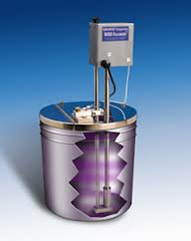7.  Oscillating Piston Viscometer

Invented at Cambridge Viscosity in 1986, this viscometer is also referred to as electromagnetic viscometer or EMV viscometer. The instrument comprises a measurement chamber and magnetically influenced piston.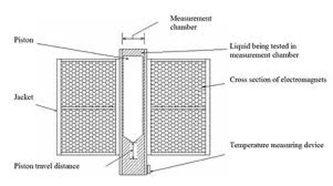Measurements are taken whereby a sample is first introduced into the thermally controlled measurement chamber where the piston resides. Electronics drive the piston into oscillatory motion within the measurement chamber with a controlled magnetic field. A shear stress is imposed on the fluid due to the piston travel and the viscosity is determined by measuring the travel time of the piston. The construction parameters for the annular spacing between the piston and measurement chamber, the strength of the electromagnetic field, and the travel distance of the piston are used to calculate the viscosity according to Newton’s law of viscosity.

8. Vibrational viscometers

The fluid viscosity is measured by the damping of an oscillating electromechanical resonator immersed in it. The resonator generally oscillates in torsion or transversely (as a cantilever beam or tuning fork). The higher the viscosity, the larger the damping imposed on the resonator. The resonator's damping may be measured by one of several methods:

1.Measuring the power input necessary to keep the oscillator vibrating at a constant amplitude. The higher the viscosity, the more power is needed to maintain the amplitude of oscillation.

2.Measuring the decay time of the oscillation once the excitation is switched off. The higher the viscosity, the faster the signal decays.

3.Measuring the frequency of the resonator as a function of phase angle between excitation and response waveforms. The higher the viscosity, the larger the frequency change for a given phase change.

9. Other rotational viscometer: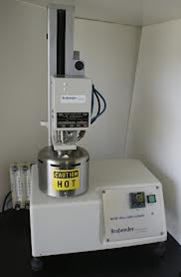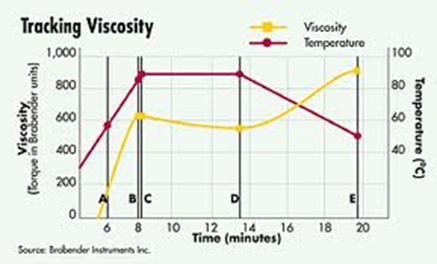There are some empirical viscometers in which a paddle, a cylinder, or bars rotate in a container, usually with large clearance between the rotating member and the wall. Eg. Brookfield viscometer, FMC consistometer, Brabender viscoamylograph